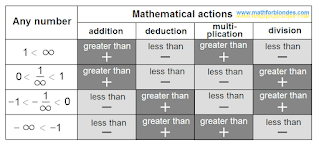## 10/17/2011

### Relativity of concept is "Any Number"

For the receipt of numerical axis does not matter, what from numbers are taken for any number: positive anymore units, positive less than units negative anymore minus units or negative less than minus units. Imposition reverse and mirror symmetries on any of these groups of numbers results in the receipt of all row of the real numbers.

From the choice of group of numbers as any number the results of mathematical actions will depend are different combinations of increase or diminishing of any number as a result of concrete mathematical action. In a table below the possible variants of concept "Any number" are marked just as there are corresponding to them fragments of numerical axis in traditionally assumed an air. For evidentness the increase of any number is doubled by a sign "+", diminishing - by the sign of "-", corresponding cells are distinguished by a different color.Relativity of concept is Any Number

As be obvious from a table, addition and deduction are mirror symmetric in relation to a point "zero". An increase and division are mirror symmetric relatively two points are points "unit" and points "zero", here reverse symmetry is mirror symmetric in relation to a point "zero". All reasoning about priority and secondaryness of mathematical actions are an error. Symmetry of mathematical actions is considered in the separate article.

Expl for blondes: Farther we will consider units of measurements and mathematical actions. More interesting things on the page "New Math".